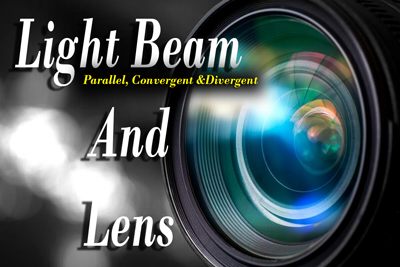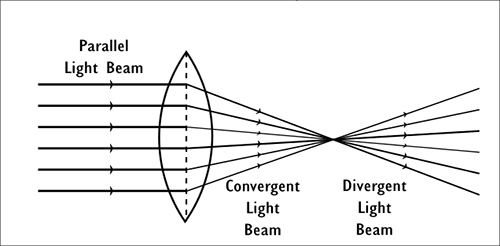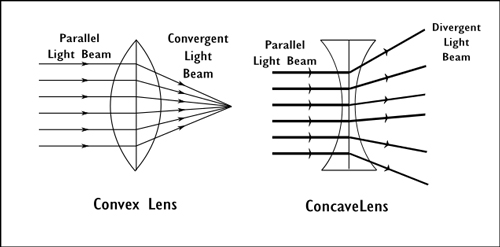Light Beam and Lenses - physics Light
11-01-2020    318 times### Rays of light

The straight line or path between two specific points in a light medium by which light travel is known as a light ray. Light rays a denoted by an arrow line that sets the direction of light. It is a vector quantity.

The set of light rays is known as a light beam. Three types of the light beam are possible

1. Parallel Light Beam: If each and every light rays in a light beam are parallel to each other then it is known as a parallel light beam. When a light source situated at a long distance then the light beams came from that source is known as a parallel beam. E.g. sunlight.
2.3. Convergent Light Beam: If light rays of a light beam coming from different directions and meet a point, then such light beam known as a convergent beam.
4. Divergent Light Beam: If light rays of a light beam coming from a single point and travel in a different direction, then such light beam known as a divergent beam of rays.

### Lens

Surround by two opposite surfaces, either both curved or one curve and another plane, a piece of transparent material (such as glass) and the homogeneous medium is known as a lens. Lenses are boldly categorised in two types

1. Convex Lens or converging Lens: If a parallel light beam receives by one side of the lens and releases a convergent light beam from another side of the lens then that lens is known as a convex lens. In these types of lenses, the middle portion is thicker than the outer side.

E.g : Looking Glass

2.3. Concave Lens or Diverging Lens: If a parallel light beam receives by one side of the lens and releases a divergent light beam from another side of the lens then that lens is known as a concave lens. In these types of lenses, the middle portion is thinner than the outer side.